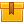# Control Charts for Variables (A8503)

## Prerequisites

This lesson is designed for participants familiar with the principles of statistical process control, the basic components of control charts, and the characteristics of a normal curve. A knowledge of basic mathematical skills is recommended.

## Description

This lesson explains how to interpret variable control charts in order to determine whether or not a process is in statistical control. The lesson introduces the concept of performance-based limits for process control and presents basic guidelines for proper sampling. In addition, the principles for interpreting control charts are presented.

## Objectives

Identify variables. Explain how the control limits for a process are related to a normal curve. Explain how the central line may be identified for mean, median, and range charts. Identify the criteria of good sampling practice. Identify a random pattern of values using the 2/3 rule. Identify three different types of non-random patterns and interpret their meaning. Explain when a non-random pattern may be a sign of process improvement.

## ContentsThis lesson explains how to interpret variable control charts in order
to determine whether or not a process is in statistical control. The
lesson introduces the concept of performance-based limits for process
control and presents basic guidelines for proper sampling. In addition,
the principles for interpreting control charts are presented.

•Control Charts for Variables

0
0 Reviews